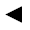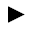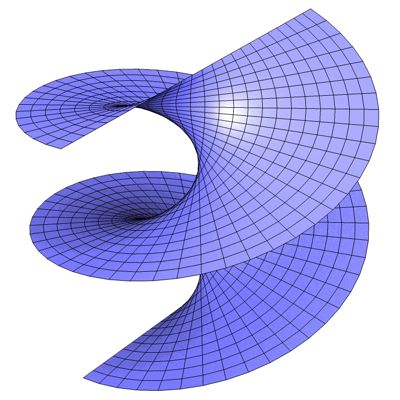﻿﻿﻿ math handbook calculator - Fractional Calculus Computer Algebra System software+++=﻿[back]

## 3.20. helicoid

[next]The helicoid [ 3,11 ] is represented by the following equations.

 x = u cos(v) 3-74 y = u sin(v) 3-75 z = cv 3-76

The constant c determines the appearance of the figure.

To represent the area, the two parameters u and v can assume the following values ​​(domain of definition), for example.

 u is an element from the set of numbers [-2.0, 2.0] v is an element of the number set [0, 2pi]

The helicoid is a half-open figure, so the domain of u can be changed in the plugin.Fig. 24